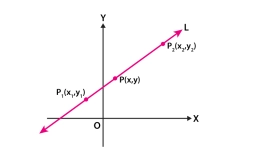# The temperature 38

The temperature has been dropping 2 degrees every hour, and the temperature at 10:00 AM was -15 degrees Fahrenheit. At what time was the temperature 0 degrees Fahrenheit?

Result

t0 = 2:30 hh:mm### Step-by-step explanation:Did you find an error or inaccuracy? Feel free to write us. Thank you!

Tips for related online calculators
Do you have a linear equation or system of equations and looking for its solution? Or do you have a quadratic equation?